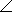# Electrical Engineering - RC Circuits - Discussion

### Discussion :: RC Circuits - General Questions (Q.No.12)

12.

The complex number 6 + j6 is equivalent to

 [A]. 645° [B]. 3645° [C]. 8.4845° [D]. 8.4890°

Explanation:

No answer description available for this question.

 Sadananda said: (Mar 13, 2012) x=a+jb |x|=sqrt(squar(a)+squar(b)) angle(a)=tan-1(a/b)

 Hanumantha said: (Jan 28, 2015) @Sadananda. Its not tan-1(a/b) its tan-1(b/a).

 Abhishek Mishra said: (Sep 26, 2015) Alpha = tan-1(b/a). Its alpha = tan-1(6/6). = tan-1(1) = 45.

 Jansi said: (Jan 26, 2016) How does that 8.48 has come? Can anyone help me in answering this?

 Malleswar Rao said: (Feb 3, 2016) Square root of (a^2+b^2) = Square root of 72 = 8.48. tan-1 (b/a) = tan-1 (6/6) = 45.

 Surya said: (Dec 28, 2016) Yeah, that's correct @Malleswar.